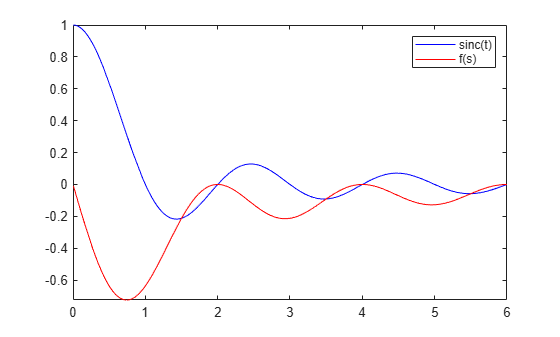# ihtrans

Inverse Hilbert transform

## Syntax

``f = ihtrans(H)``
``f = ihtrans(H,transVar)``
``f = ihtrans(H,var,transVar)``

## Description

example

````f = ihtrans(H)` returns the inverse Hilbert transform of symbolic function `H`. By default, the independent variable is `x` and the transformation variable is `t`.```

example

````f = ihtrans(H,transVar)` uses the transformation variable `transVar` instead of `t`.```

example

````f = ihtrans(H,var,transVar)` uses the independent variable `var` and the transformation variable `transVar` instead of `x` and `t`, respectively. If all input arguments are arrays of the same size, then `ihtrans` acts element-wise.If one input is a scalar and the others are arrays of the same size, then `ihtrans` expands the scalar into an array of the same size.If `f` is an array of symbolic expressions with different independent variables, then `var` must be a symbolic array with elements corresponding to the independent variables. ```

## Examples

collapse all

Compute the inverse Hilbert transform of `cos(x)`. By default, the inverse transform returns a function of `t`.

```syms x; f = cos(x); H = ihtrans(f)```
`H = $-\mathrm{sin}\left(t\right)$`

Compute the inverse Hilbert transform of the `sinc` function, which is equal to `sin(πt)/πt`. Express the result as a function of `s`.

```syms H(t) f(s); H(t) = sinc(t); f(s) = ihtrans(H,s)```
```f(s) =  $\frac{\frac{\mathrm{cos}\left(\pi s\right)}{s}-\frac{1}{s}}{\pi }$```

Plot the `sinc` function and its inverse Hilbert transform.

```fplot(H(t),[0 6],'b') hold on fplot(f(s),[0 6],'r') legend('sinc(t)','f(s)')```Create a sine wave with a positive frequency in real space.

```syms A x t u; assume([x t],'real') H = A*sin(2*pi*10*t + 5*x)```
`H = $A \mathrm{sin}\left(5 x+20 \pi t\right)$`

Apply a 90-degree phase shift to the positive frequency component using the inverse Hilbert transform. Specify the independent variable as `x` and the transformation variable as `u`, respectively.

`f = ihtrans(H,x,u)`
`f = $A \mathrm{cos}\left(5 u+20 \pi t\right)$`

Now create a complex signal with negative frequency. Apply a –90-degree phase shift to the negative frequency component using the inverse Hilbert transform.

`Z = A*exp(-1i*10*t)`
`Z = $A {\mathrm{e}}^{-10 t \mathrm{i}}$`
`f = ihtrans(Z)`
`f = $-A {\mathrm{e}}^{-10 u \mathrm{i}} \mathrm{i}$`

Create a real-valued signal $f\left(s\right)$ with two frequency components, 60 Hz and 90 Hz.

```syms s f(x) F(t) f(s) = sin(2*pi*60*s) + sin(2*pi*90*s)```
`f(s) = $\mathrm{sin}\left(120 \pi s\right)+\mathrm{sin}\left(180 \pi s\right)$`

Calculate the corresponding analytic signal $F\left(t\right)$ using the inverse Hilbert transform.

`F(t) = ihtrans(f(s),t) + 1i*f(t)`
`F(t) = $\mathrm{cos}\left(120 \pi t\right)+\mathrm{cos}\left(180 \pi t\right)+\mathrm{sin}\left(120 \pi t\right) \mathrm{i}+\mathrm{sin}\left(180 \pi t\right) \mathrm{i}$`

Calculate the instantaneous frequency of $F\left(t\right)$ using

`${f}_{instant}\left(t\right)=\frac{1}{2\pi }\frac{d\varphi \left(t\right)}{dt},$`

where $\varphi \left(t\right)=\mathrm{arg}\left[F\left(t\right)\right]$ is the instantaneous phase of the analytic signal.

```InstantFreq(t) = diff(angle(F(t)),t)/(2*pi); assume(t,'real') simplify(InstantFreq(t))```
`ans = $75$`

## Input Arguments

collapse all

Input, specified as a symbolic expression, symbolic function, symbolic vector, or symbolic matrix.

Independent variable, specified as a symbolic variable, symbolic vector, or symbolic matrix. This variable is usually in the time domain. If you do not specify the variable, then `ihtrans` uses `x` by default. If `H` does not contain `x`, then `ihtrans` uses the function `symvar` to determine the independent variable.

Transformation variable, specified as a symbolic variable, symbolic vector, or symbolic matrix. This variable is in the same domain as `var`. If you do not specify the variable, then `ihtrans` uses `t` by default. If `t` is the independent variable of `H`, then `ihtrans` uses the transformation variable `u`.

## Output Arguments

collapse all

Inverse Hilbert transform of the input function `H`. The output `f` is a function of the variable specified by `transVar`.

When `ihtrans` cannot transform the input function, it returns an unevaluated call. To return the original expression, apply the Hilbert transform to the output by using `htrans`.

collapse all

### Inverse Hilbert Transform

The inverse Hilbert transform f = f(t) of the expression H = H(x) with respect to the variable x at point t is

`$f\left(t\right)=\frac{1}{\pi }\text{p}\text{.v}\text{.}\underset{-\infty }{\overset{\infty }{\int }}\frac{H\left(x\right)}{x-t}dx.$`

Here, p.v. represents the Cauchy principal value of the integral. The function H(x) can be complex, but x and t must be real.

## Tips

• To compute the Hilbert transform, use `htrans`. The inverse Hilbert transform of a function is equal to the negative of its Hilbert transform.

• For a signal in the time domain, the inverse Hilbert transform applies a 90-degree phase shift to negative frequencies of the corresponding Fourier components. It also applies a –90-degree phase shift to positive frequencies.

• A real-valued signal `b` is the harmonic conjugate of its inverse Hilbert transform `a = ihtrans(b)`. The inverse Hilbert transform `a = real(z)` and the signal `b = imag(z)` form the analytic signal `z = a + 1i*b`.